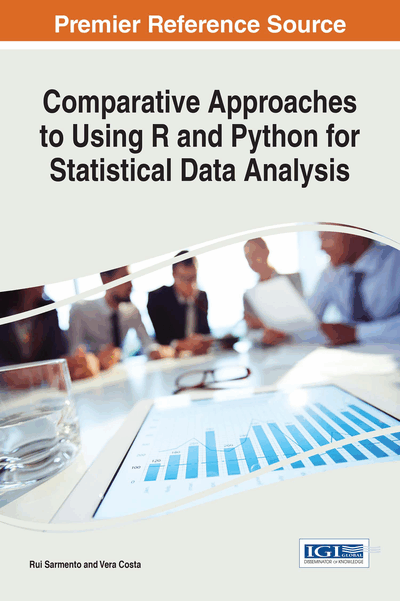# Statistics

Copyright: © 2017 |Pages: 31
DOI: 10.4018/978-1-68318-016-6.ch001
OnDemand PDF Download:
Available
\$37.50
No Current Special Offers

## Abstract

Statistics is a set of methods used to analyze data. This chapter presents the main concepts used in statistics, learning from data is one of the most critical challenges. In general, we can say that statistic based on the theory of probability, provides techniques and methods for data analysis, which help the decision-making process in various problems where there is uncertainty.
Chapter Preview
Top

## Variables, Population, And Samples

In statistical analysis, “variable” is the common characteristic of all elements of the sample or population to which is possible to attribute a number or category. The values of the variables vary from element to element.

### Types of Variables

Statistical variables can be classified as categorical variables or numerical variables.

Categorical variables have values that describe a “quality” or “characteristic” of a data unit, like “which type” or “which category”. Categorical variables fall into mutually exclusive (in one category or another) and exhaustive (include several possible options) categories. Therefore, categorical variables are qualitative variables and tend to be represented by a non-numeric value. Categorical variables may be further described as (Marôco, 2011):

• Nominal: The data consist of categories only. The variables are measured in discrete classes, and it is not possible to establish any qualification or ordering. Standard mathematical operations (addition, subtraction, multiplication, and division) are not defined when applied to this type of variable. Gender (male or female) and colors (blue, red or green) are two examples of nominal variables.

• Ordinal: The data consist of categories that can be arranged in some exact order according to their relative size or quality, but cannot be quantified. Standard mathematical operations (addition, subtraction, multiplication, and division) are not defined when applied to this type of variable. For example, social class (upper, middle and lower) and education (elementary, medium and high) are two examples of ordinal variables. Likert scales (1-“Strongly Disagree”, 2-“Disagree”, 3-“Undecided”, 4-“Agree”, 5-“Strongly Agree”) are ordinal scales commonly used in social sciences.

Numerical variables have values that describe a measurable quantity as a number, like “how many” or “how much”. Therefore, numeric variables are quantitative variables. Numeric variables may be further described as:

• Discrete: The data is numerical. Observations can take a value based on a count of a set of distinct integer values. A discrete variable cannot take the value of a fraction of one value and the next closest value. The number of registered cars, the number of business locations, and the number of children in a family, all of which measured as whole units (i.e. 1, 2, or 3 cars) are some examples of discrete variables.

• Continuous: The data is numerical. Observations can take any value between a particular set of real numbers. The value given to one observation for a continuous variable can include values as precise as possible with the instrument of measurement. Height and time are two examples of continuous variables.

## Complete Chapter List

Search this Book:
Reset